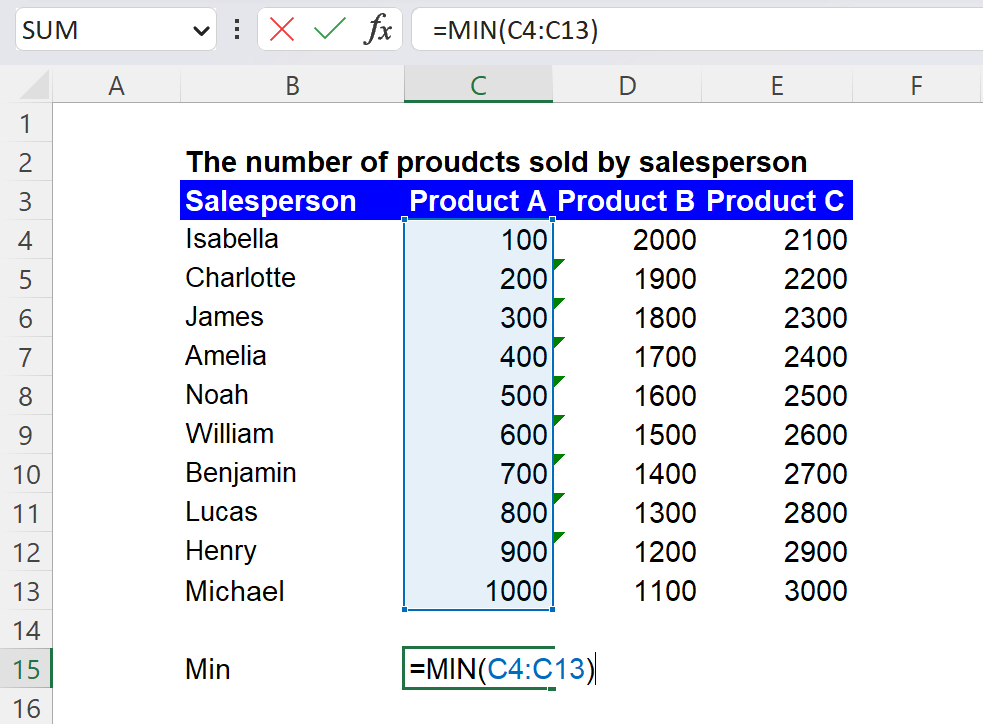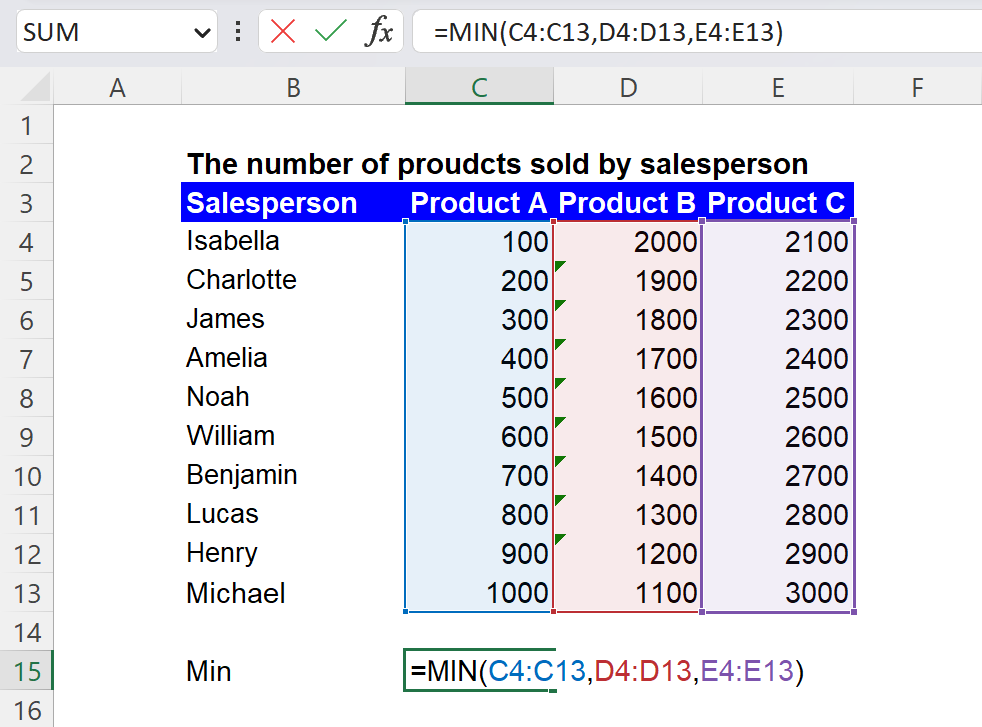Excel Formulas

# MIN Function in Excel: Explained

In this article, you will learn how to use the MIN formula in Excel.

## What is the MIN formula in Excel?

In Excel, the MIN function returns the smallest number in a dataset.

## When is the MIN function beneficial in Excel?

The MIN function in Excel can be beneficial in various situations where you need to find the smallest number in a set of values. Some examples include:

1. Finding the lowest score or minimum grade in a class or group of students.
2. Identifying the lowest price or smallest value of a stock or commodity in a certain period.
3. Determining the earliest date or the shortest time in a list of events or tasks.

The MIN function can be beneficial when you have a large amount of data and need to quickly identify the smallest value in a specific range of cells. It can also be helpful when you need to compare values from multiple ranges and determine which one has the smallest value.

## How to use the MIN function in Excel

To use the MIN function in Excel, you need to enter the function into a cell with the following syntax:

<pre><code>=MIN(number1, [number2], ...)</code></pre>

"number1" is the first number in the set of values you want to find the smallest of.

"number2" and any other numbers in the function are optional additional numbers in the set.

The MIN formula returns the lowest among all ranges.

Note: Empty cells, logical values, or text in the selected cells or arrays are ignored. The MIN formula returns zero if the selected ranges contain no numeric values.

For example, if you want to find the smallest number in a range of cells from C4 to C13, you would enter the following formula into a cell:

``=MIN(C4:C13)``

The formula should return 100, the most minor figure in the selected range.How to use the MIN formula with a single range in Excel

You can select multiple ranges in the formula, as below. The formula should return a value of 100, the lowest value in the selected cells.

``=MIN(C4:C13,D4:D13,E4:E13)``How to use the MIN function with multiple ranges in Excel

Or, you can select the entire array as follows:

``=MIN(C4:E13)``

You can also use the MIN function to find the smallest number in a set of numbers that you enter manually. For example, if you want to find the smallest number among the numbers 2, 5, and 8, you would enter the following formula into a cell:

``=MIN(2,5,8)``

## What is the difference between the MIN and MINIFS formulas in Excel?

The MIN and MINIFS functions in Excel are used to find the smallest number in a set of values, but they have some key differences. The MIN function finds the smallest number in a group of values, regardless of other criteria. On the other hand, the MINIFS function finds the smallest number in a set of values based on one or more conditions.  For example, suppose you have a range of cells with values and another range of cells with corresponding categories. In that case, you could use the MINIFS function to find the smallest value in the first range but only among the values corresponding to a specific category in the second range.

## Analyze your live financial data in a snap in Google Sheets

Are you learning this formula to visualize financial data, build a financial model, or conduct financial analysis? In that case, LiveFlow may help you automate manual workflows, update numbers in real-time, and save time. You can access various financial templates on our website, from the simple Income Statement to Multi-Currency Consolidated Financial Statement. Are you interested in this product but are an Excel user? That’s not a problem at all. You can connect Google Sheets to Excel quickly.